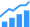# Maximizing Edge AI Performance

Simple steps to make sure you get the fastest inferences.Inference of convolutional neural network models is algorithmically straightforward, but to get the fastest performance for your application there are a few pitfalls to keep in mind when deploying. A number of factors make efficient inference difficult, which we will first step through before diving into specific solutions to address and resolve each. By the end of this article, you will be armed with four tools to use before building your system.

### Why accelerate convolutional layers?

Broadly speaking, convolutions are all about sliding a function over something else. In the context of image data, we slide a window over pixels with three channels (RGB) and apply the same function on each window.

In a convolutional layer of a CNN, the function performed in every window is actually an element-wise multiplication with a matrix (necessarily of equal size) of fixed values called a filter. A set of multiple filters is also known as a convolutional kernel. The number of filters in this kernel will ultimately be the number of channels that the layer will output.Fig. 2: In a convolutional layer, the actual function we are convolving is a series of element-wise matrix multiplications with different filters. Note: Each mathematical operation is actually a fused multiply and add (FMA) operation, also known as a ‘tensor op’.

## Use fast matrix multiplication algorithms

The first and biggest challenge with CNN inference is that each layer requires a massive amount of matrix multiplies, as mentioned above. The number of operations scale with the size of the image, as well as the number of filters in each layer. While there’s no way to avoid these computations, specialized inference solutions have hardware for fast matrix multiplication algorithms such as the Winograd transformation. On common 3×3 convolutional kernels, such transformations can have the effect of reducing the number of operations needed by 2.25x! Therefore, the first and most general optimization you can make is to ensure that your deployment solution is able to leverage the advantages that fast matrix multiplication algorithms like Winograd can provide. For example, dedicated SoCs like Flex Logix’s InferX X1 have circuitry built in that can dynamically perform the transformations necessary for Winograd multiplication.

## Quantize to lower precision data types

Just as the number of multiplications can vary dramatically between layers, so too does the amount of data that needs to be passed between layers. This data is known as activation energies, or activations. Inherently neural networks are approximations, and once a function has been trained in FP32 or FP16, the extra precision that these data types provide is unnecessary for inference. The process of changing the data type of a CNN is known as quantization. In common frameworks like PyTorch and TensorflowLite, quantization to INT8 can be accomplished after training with a tiny fraction of the data required for training, and only a few extra lines of code. The benefit of quantizing for inference can result in an immediate 2x improvement in latency over inference even in FP16!

## Choose hardware with flexibility

Next up, as inference proceeds through a CNN, each layer does a different convolution from the previous layer. Whether it’s changing the window size of the kernel or using a different number of filters, the operations that mold and shape the activations end up having different ratios of memory access to computation. An early layer may have many more computations relative to the amount of memory it requires, whereas a middle layer will be operating on a very large activation data but only perform a fraction of the computations. Inherently, then, an architecture that can adapt to these changing memory and computation access patterns will have an advantage over one that does not. For example, the InferX X1 leverages Flex Logix’s eFPGA technology to dynamically reconfigure between layers to maintain an optimal datapath throughout inference. So, when looking to deploy, choose an architecture that can adapt.

## Streaming data

Lastly, when training models, in a process known as backwards propagation, much information is generated to update the weights of the model based on each piece of training data. One way to cut down the amount of memory bandwidth required is to ‘batch’ the data and sum up the different changes to these weights over that set of data. In the context of inference, the approach of batching and calculating multiple inferences in parallel, going layer by layer can also improve throughput, but at the cost of latency. For example, in realtime applications, you will have to wait for enough data to come in before starting, and with some hardware, instead of using all the processing elements on a single job, you end up splitting the resources to process multiple inferences in parallel. If the fastest possible inferences is a concern for your application, remember to infer on a batch size of 1.

## Conclusion

Faster inference for real-time applications opens up new design possibilities and can ultimately save you and your customers not just time, but also money. As this article highlights, now you have a template you can apply to improve inference performance in your end application, whether that be for medical imaging, factory automation, ADAS, or something else entirely! Just remember these four key tools: 1) make sure you’re taking advantage of fast matrix multiplication algorithms, 2) quantize to INT8, 3) deploy on flexible hardware, and 4) use batch=1 for real-time applications. Leveraging these tools will ensure you get the fastest inference possible for your applications.